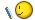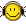# MP3TAG Maths

I have a formula that i would like to use with mp3 tag, but i can't seem to get it to work..

I am a DJ and keep my files tagged nicely with mp3 tag and it does a brill job.. what i want to do tho is to calculate a percentage increase/decrease from a bpm field (handy when mixing)..

the formula in excel is (130-%bpm%)/%bpm% then changed to a percentage (*100) (i use mix meister bpm analyser to calculate the bpm then this will tell me the percentage i have to increase the track to mix at 130bpm - lazy i am !)

i can't get this to work in mp3 tag as i think it rounds numbers or removes decimal point

i want it to create a new field or place it in the comment field as something like +1.55%

anyone help pls ?

Jon.

the only way i have found to do this so far is as follows

multiply the bpm by 100000 into the comment field
subtract 13000000 from the comment field
divide the comment by the bpm field (original value) again into the comment field
divide the comment by 1000 into the genre field (i don't use these fields for the dj songs currently)
then its the genre field + '.' + the right 3 of the comment field to give me the value as something like 1.560

long winded - so any genius got a more simple way would be appreciatedJon.

(ok i found i can just type temp1 and temp2 fields so i don't have to corrupt the comment and genre fields ! )It does seem like mp3tag rounds down all decimal numbers before they are used in a function. To test, I set the bpm filed of one of my songs to 80.9. The function \$mul(%bpm%,100) returned 8000 as the answer. If you can deal with Whole number percentages, you can use this function without having to touch any other tags:

1. Go to Convert > Actions and make a new Action Group.

2. Make a new Action in that group, "Format value"

3. For "Field:", put COMMENT. For Format string, put the function:

\$div(\$sub(13000,\$mul(%bpm%,100)),%bpm%)

1. Run the action Group on all your songs.

That's the best I can come up with for now. I'll peck through the help documents and see what I find.

To find some useful hints about manipulating BPM within Mp3tag you may do a google search
"site:anytag.de bpm"

For bpm values with two decimals you may try an expression like this:
\$div(\$mul(100,\$sub(13000,\$regexp(%bpm%,'[.,]',\$1))),\$regexp(%bpm%,'[.,]',\$1))

A given bpm value of '123.45' will result to '5' percent. (5,30579181855002025111381125961)
A given bpm value of '133.75' will result to '-2' percent. (-2,80373831775700934579439252336)

Mp3tag's integer math cuts off the fractional part, so you may tweak the expression to work with promille instead of percent to get a better granularity.

DD.20090205.0639.CET

actually thats quite brilliant as if does everything i did in several lines in one if i change it a bit.. one problem left tho is this - i have managed to get the result as a number ie 1560 or 0650 and can change that with string operators and the number function to read 1.540 or 0.650 for example but the problem comes with the odd tag where the number is just returned as -7 which should read -0.07 but it the number function gets the minus in the wrong place so i get 0.-7 for example not sure if this will mean anything to anyone ?

Jon.

ok, my expression is this :-

``````\$div(\$mul(100000,\$sub(13000000,\$regexp(\$add(\$mul(%bpm%,100000),\$mul(1000,\$right(%bpm%,2))),'[.,]',\$1))),\$regexp(\$add(\$mul(%bpm%,100000),\$mul(1000,\$right(%bpm%,2))),'[.,]',\$1))
``````

this gives me results as follows :-

2354
1570
549
-115
-7
-1492

the positive numbers are fine as is the -1492 number but the other 2 when i use the number function to make if a fixed length messes it up and i get 0-115 or 000-7 etc.. so the number function is getting it wrong. If there is an expression anyone can think of that would convert the minus numbers properly to -0115 or -0007 for example i would be grateful and my life would be complete (till the next problem - lol)

Jon.

Try this ...

\$ifgreater(\$regexp(%BPM%,'[.,]',),13000, '-'\$right('000000'\$div(\$mul(-100000,\$sub(13000,\$regexp(%BPM%,'[.,]',))), \$regexp(%BPM%,'[.,]',)),6), '+'\$right('000000'\$div(\$mul(100000,\$sub(13000,\$regexp(%BPM%,'[.,]',))), \$regexp(%BPM%,'[.,]',)),6))
... or this ... \$ifgreater(\$regexp(%BPM%,'[.,]',),13000,'-','+') \$right('000000'\$regexp(\$div(\$mul(100000,\$sub(13000,\$regexp(%BPM%,'[.,]',))), \$regexp(%BPM%,'[.,]',)),'^-',),6)
You will get results like ...
127.68/130.00: '+001649'
140.00/130.00: '-007142'

Afterwards you may format the result e. g. to '-7.142%' ...

\$div('-007142',1000)'.'\$right('-007142',3)'%'

DD.20090218.1152.CET

brilliant.. the only thing i have changed is instead of using the \$div i used the string operator to add the decimal point and add the percent - hence keeping the +/- sign and making the -000007 work properly.
its actually way more accurate than any dj decks percentage control and i'll test its accuracy for the mix sat nightthis all assumes the bpm field is accurate (i use mix meister bpm analyzer for this)

cheers